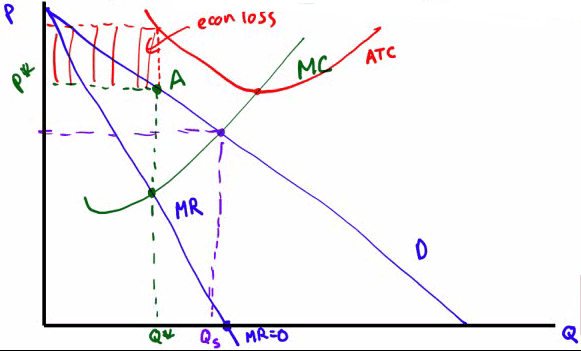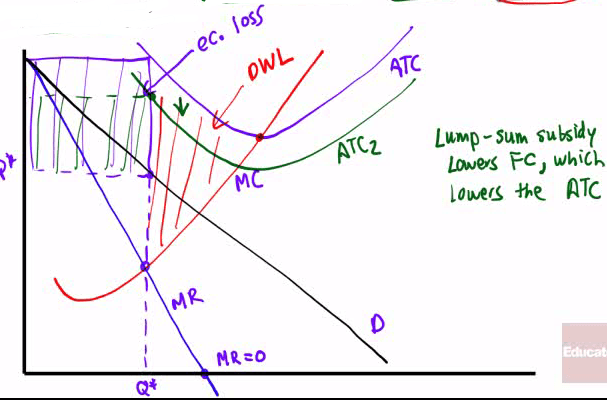# Welfare Effects of Monopoly

• Monopoly vs. Perfect Competition (Surplus)

• Assume a downward sloping demand curve for both monopoly and prefect competition with a constant MC as well as ATC

• In a monopoly, the marginal revenue will be below the demand curve.

• Consumer surplus is reduced and deadweight loss (DWL) is created

• Graph• Summary

• By holding output level below the level at which marginal cost is equal to the market price, a monopolist increases profits but decreases consumer surplus

• Mutually beneficial transactions do not occur, but a monopolist is (naturally) looking out for its own interests.

• Perfectly competitive firms also profit-maximize, but they produce where P = MC, which is also MR = MC

• Monopolists produce at MR = MC, but P > MC

• This creates deadweight loss or DWL

# Public Ownership of Monopolies

• Many countries opt for public ownership of natural monopolies (economies of scale)

• In theory, the government can set prices based on efficiency (P = MC) rather than profit maximization (MR = MC)

• In practice, publicly owned firms have less incentives to keep costs down or offer high quality

• Electricity, local phone service, water and gas are examples of regulated monopolies

• Should the government regulate cable TV?

# Unregulated vs. Regulated Natural Monopoly

• Assume a demand curve for both situations with a demand intersecting ATC on downward-sloping portion

• Unregulated monopoly charges MR = MC (econ profit)

• Regulated monopoly charges (normal profit)# Monopoly Practice Problem• Assume an unregulated monopoly.

• The monopolist's quantity produced

• where MR = MC, at point c

• The monopolist's price

• above point c, at point a

• The economic profit of the monopolist

• between ac and the y-axis

• The area of deadweight loss

• between ac and demand

• Assume the monopolist can perfectly price discriminate

• The quantity produced

• where MR = MC = D, at point f

• The total revenue of the monopolist

• asking for revenue, not profit

• Assume a monopolist is regulated to maximize total surplus

• The socially efficient quantity

• socially efficient = allocatively efficient = when P equals MC = maximum of consumer surplus and producer surplus

• The consumer surplus at the socially efficient quantity

• Is the monopolist facing regulation earning a positive economic profit, earning zero economic profit, or incurring a loss? Explain your answer.

• at point f, where price = marginal cost = average total cost

• Accounting profit = TR - TC = Q* (P - ATC) = 0

• Is point f in the elastic, inelastic, or unit elastic portion of the demand curve? Explain.

• MR > 0, elastic

• MR < 0, inelastic

• MR = 0 , unit elastic

# More Monopoly Practice Problem

• Zachrail, the only provider of train services between two cities, is currently incuring economic losses

• Show Zachrail's loss-minimizing price and quantity

• loss-minimizing = profit-maximizing

• the point on demand curve above the point where MR = MC

• Show the area of economic loss

• the point on ATC curve above the point where MR = MC
• Identify the allocatively efficient quantity

• the point where D = MC• If Zachrail raised the price above the profit-maximizing price, would total revenue increase, decrease or not change? Explain.

• If elastic, P↑, TR↓

• If elastic, P↓, TR↑

• If inelastic, P↑, TR↑

• If inelastic, P↓, TR↓

• Would a per-unit tax or per-unit subsidy be advisable in this situation if the goal is to produce at the allocatively efficient point? Explain why.

• Explanation: lead towards allocatively efficient point• Assume instead that a lump-sum subsidy is provided to Zachrail. In the short run, will deadweight loss increase, decrease of not change? Will Zachrail's economic losses increase, decrease or not change?

• Lump-sum subsidy lowers FC, which lowers the ATC

• Answer: the deadweight loss will not be changed, the losses will decrease